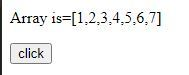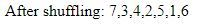# How to shuffle an array using JavaScript ?

Shuffling an array or a list means that we randomly re-arranging the content of that structure. To shuffle an array we will use the following algorithm:

Algorithm:

 `function` `shuffleArray(array) { ` `   ``for` `(``var` `i = array.length - 1; i > 0; i--) {  ` `   `  `       ``// Generate random number  ` `       ``var` `j = Math.floor(Math.random() * (i + 1)); ` `                   `  `       ``var` `temp = array[i]; ` `       ``array[i] = array[j]; ` `       ``array[j] = temp; ` `   ``} ` `       `  `   ``return` `array; ` `} `

Example:

 ` ` `<``html``> ` ` `  `<``head``> ` `    ``<``title``>Shuffle array ` ` ` ` `  `<``body``> ` `    ``<``p``>Array is=[1, 2, 3, 4, 5, 6, 7] ` `     `  `    ``<``button` `onclick``=``"show()"``> ` `        ``click ` `    `` ` `     `  `    ``<``script``> ` ` `  `        ``// Fucntion to shuffle the array content ` `        ``function shuffleArray(array) { ` `            ``for (var i = array.length - 1; i > 0; i--) { ` ` `  `                ``// Generate random number  ` `                ``var j = Math.floor(Math.random() * (i + 1)); ` ` `  `                ``var temp = array[i]; ` `                ``array[i] = array[j]; ` `                ``array[j] = temp; ` `            ``} ` ` `  `            ``return array; ` `        ``} ` ` `  `        ``// Function to show the result ` `        ``function show() { ` `            ``var arr = [1, 2, 3, 4, 5, 6, 7] ` `            ``var arr1 = shuffleArray(arr) ` ` `  `            ``document.write("After shuffling: ", arr1) ` `        ``} ` `    `` ` ` ` ` `  ` `

Output:

• Before Clicking the Button:• After Clicking the button:My Personal Notes arrow_drop_upCheck out this Author's contributed articles.

If you like GeeksforGeeks and would like to contribute, you can also write an article using contribute.geeksforgeeks.org or mail your article to contribute@geeksforgeeks.org. See your article appearing on the GeeksforGeeks main page and help other Geeks.

Please Improve this article if you find anything incorrect by clicking on the "Improve Article" button below.